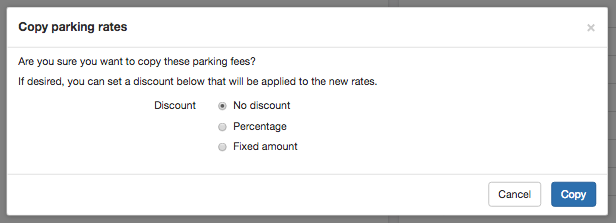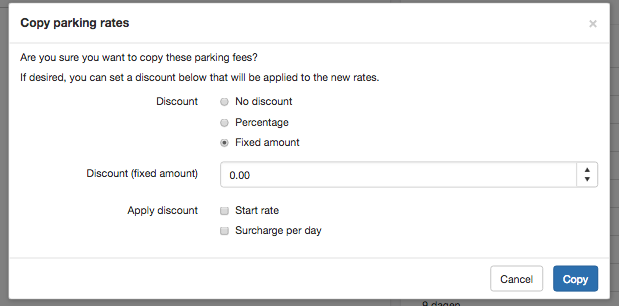By copying a price list you can use an existing price list as a basis for a new price list. The copy price list function also offers some tools to calculate new rates based on an existing price list, for example when you want to adjust your price with a percentage or fixed amount.

Where can you find the copy price list function?1. Select the menu option "Settings".

2. Then you press "Parking fees".

3. Select the price list you want to copy from the list of price lists.

4. Via the "..." in the top of the right, you will find the menu item to copy your price list.

Copy possibilities :1. No discount means that the source price list as it is currently being copied.

2. Percentage applies the discount as a percentage of the price.

3. Fixed amount applies the discount as a fixed amount of the price.

Apply discount percentage1. Discount (procent): The percentage of the discount.A surcharge can also be applied by applying a negative discount               percentage (for example -10%).

2. Apply discount to: Start rate calculate the discount or surcharge by on the starting rate column of the price list. Via the           Surcharge per day option you can apply the discount to the price per day column of the price list.

Apply fixed discount amount.1. Discount (fixed amount): The amount of the discount.

A surcharge can also be applied by applying a negative discount percentage (for example -10%).

2.    Apply discount to:

Start rate calculate the discount or surcharge by on the starting rate column of the price list. Via the Surcharge per day           option you can apply the discount to the price per day column of the price list.